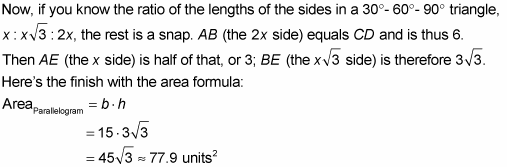##### Geometry Essentials For Dummies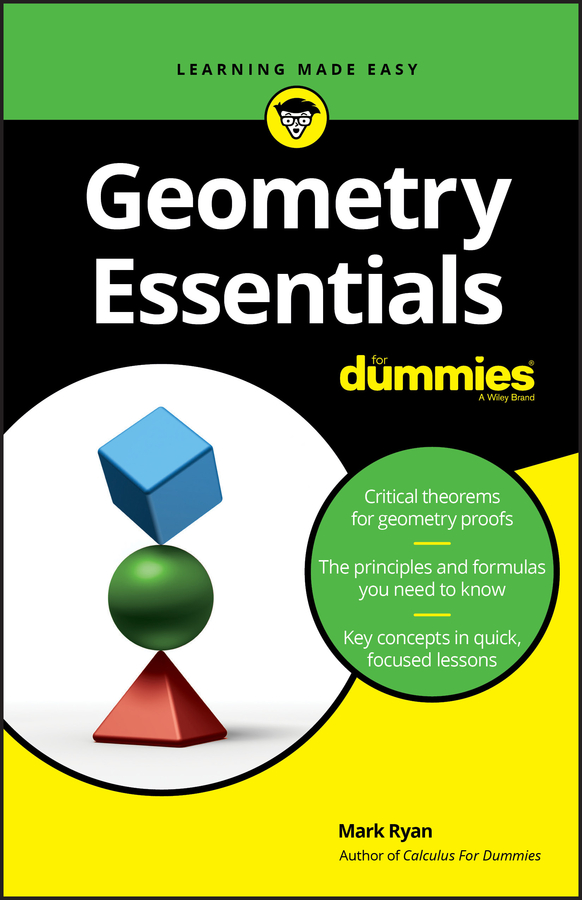The area formulas for the parallelogram, kite, and trapezoid are based on the area of a rectangle. The following figures show you how each of these three quadrilaterals relates to a rectangle, and the following list gives you the details: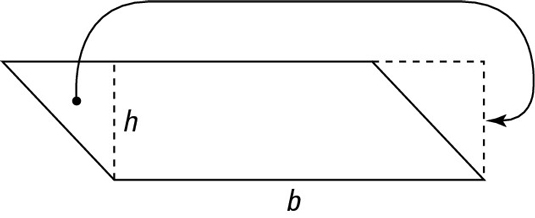• Parallelogram: In the above figure, if you cut off the little triangle on the left and fill it in on the right, the parallelogram becomes a rectangle (and the area obviously hasn’t changed). This rectangle has the same base and height as the original parallelogram. The area of the rectangle is base times height, so that formula gives you the area of the parallelogram as well. You can try this yourself by cutting out a paper parallelogram and snipping off the triangle as shown in the above figure.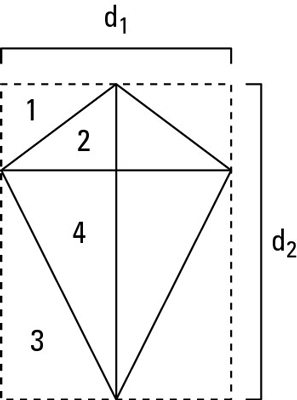• Kite: The above figure shows that the kite has half the area of the rectangle drawn around it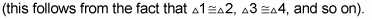You can see that the length and width of the large rectangle are the same as the lengths of the diagonals of the kite.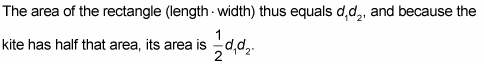• Trapezoid: If you cut off the two triangles and move them as shown in the following figure, the trapezoid becomes a rectangle. This rectangle has the same height as the trapezoid, and its base equals the median (m) of the trapezoid. Thus, the area of the rectangle (and therefore the trapezoid as well) is median times height.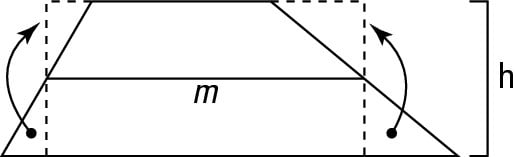The key for many quadrilateral area problems is to draw altitudes and other perpendicular segments on the diagram. Doing so creates one or more right triangles, which allows you to use the Pythagorean Theorem or your knowledge of special right triangles, such as the 45°- 45°- 90° and 30°- 60°- 90° triangles.

Here’s an example: Find the area of parallelogram ABCD in the following figure.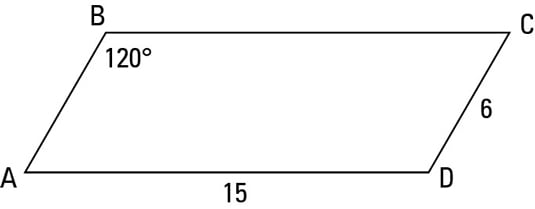When you see a 120° angle in a problem, a 30°- 60°- 90° triangle is likely lurking somewhere in the problem. (Of course, a 30° or 60° angle is a dead giveaway of a 30°- 60°- 90° triangle.) And if you see a 135° angle, a 45°- 45°- 90° triangle is likely lurking.

To get started, draw in the height of the parallelogram straight down from B to base segment AD to form a right triangle as shown in the following figure.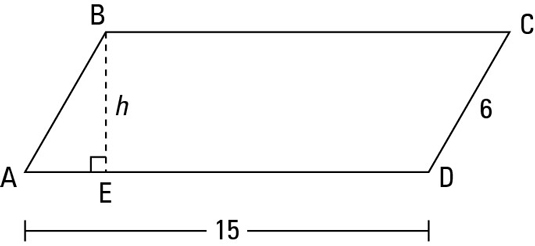Consecutive angles in a parallelogram are supplementary. Angle ABC is 120°, so angle A is 60° and triangle ABE is thus a 30°- 60°- 90° triangle.